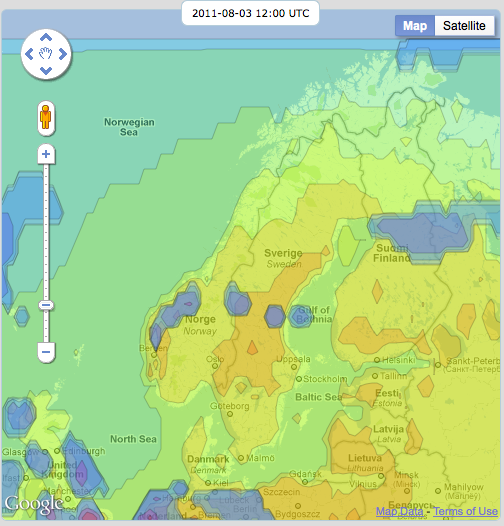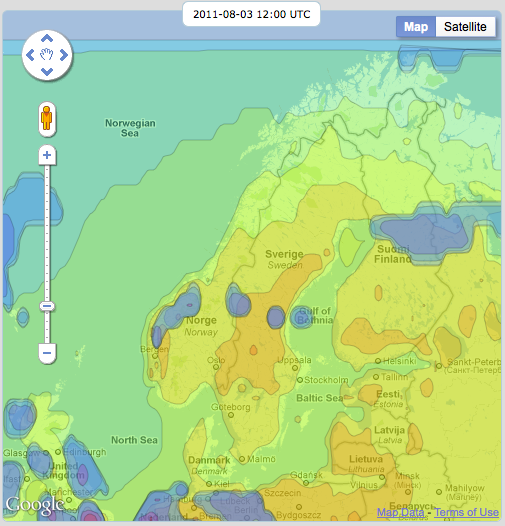# Improving Google Maps polygons with b-splines

Posted by Johan on Saturday, July 30, 2011

Google Maps is great, you get an extremely nice background map for free. I know that there are alternatives (Bing, OpenLayers, etc) out there but since I’m running Google App Engine it seems easier to go Google all the way.

I’m plotting polygons and polylines (that’s what weather is about) and it works great but my input data is kind of sparse so the polygons look very rough.

To improve them I’m using b-splines. Found a very nice article here. I just changed the javascript so it works with lat/lon-arrays and the output is an array of google.maps.LatLng.

``````function bspline(lats, lons) {
var i, t, ax, ay, bx, by, cx, cy, dx, dy, lat, lon, points;
points = [];
// For every point
for (i = 2; i < lats.length - 2; i++) {
for (t = 0; t < 1; t += 0.2) {
ax = (-lats[i - 2] + 3 * lats[i - 1] - 3 * lats[i] + lats[i + 1]) / 6;
ay = (-lons[i - 2] + 3 * lons[i - 1] - 3 * lons[i] + lons[i + 1]) / 6;
bx = (lats[i - 2] - 2 * lats[i - 1] + lats[i]) / 2;
by = (lons[i - 2] - 2 * lons[i - 1] + lons[i]) / 2;
cx = (-lats[i - 2] + lats[i]) / 2;
cy = (-lons[i - 2] + lons[i]) / 2;
dx = (lats[i - 2] + 4 * lats[i - 1] + lats[i]) / 6;
dy = (lons[i - 2] + 4 * lons[i - 1] + lons[i]) / 6;
lat = ax * Math.pow(t + 0.1, 3) + bx * Math.pow(t + 0.1, 2) + cx * (t + 0.1) + dx;
lon = ay * Math.pow(t + 0.1, 3) + by * Math.pow(t + 0.1, 2) + cy * (t + 0.1) + dy;
points.push(new google.maps.LatLng(lat, lon));
}
}
return points;
}
``````

There are some more things that you have to do, the original arrays have to be extended by adding the first 2 elements at the back and the last 2 at the front. Or else the polygon will look chopped.

Also the first point may have to be added at the end to close a polyline, a polygon will close itself.

This is what the picture looks like without b-splines:And with b-splines:There are some problems with incomplete polygons (polylines that enter and exit the area) but by skipping the first and last point they look ok.

Next step is to use Thrift for communication instead of JSON. Don’t know if it will actually make any difference but I have promised myself to learn either Google Protocol Buffers or Thrift.